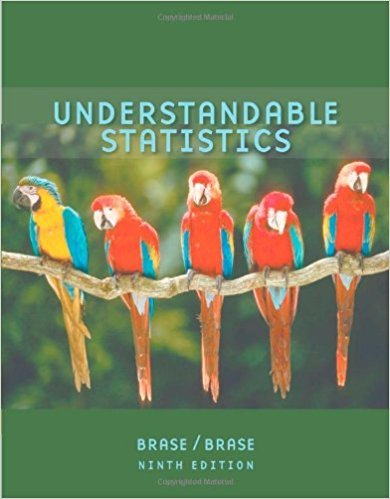×
×

# Critical Thinking An agricultural study is comparing theISBN: 9780618949922 213

## Solution for problem 7 Chapter 1.3

Understandable Statistics | 9th Edition

• Textbook Solutions
• 2901 Step-by-step solutions solved by professors and subject experts
• Get 24/7 help from StudySoup virtual teaching assistantsUnderstandable Statistics | 9th Edition

4 5 1 308 Reviews
18
3
Problem 7

Critical Thinking An agricultural study is comparing the harvest volume of two types of barley. The site for the experiment is bordered by a river. The field is divided into eight plots of approximately the same size. The experiment calls for the plots to be blocked into four plots per block. Then, two plots of each block will be randomly assigned to one of the two barley types. Section 1.3 Introduction to Experimental Design 27 Two blocking schemes are shown below, with one block indicated by the white region and the other by the grey region. Which blocking scheme, A or B, would be best? Explain. 28 Chapter 1 GETTING STARTED Scheme A River Scheme B R

Step-by-Step Solution:
Step 1 of 3

STAT 110: Notes for Week of 10/17/16  Chapter 21 o p = population parameter. o p = sample proportion/percentage. Estimates the parameter.  p = (number of successes in the sample/n) o μ = population mean/average. o x = sample mean/average. Estimates the population mean/average. o A statistic (point estimate/p)...

Step 2 of 3

Step 3 of 3

##### ISBN: 9780618949922

Unlock Textbook Solution